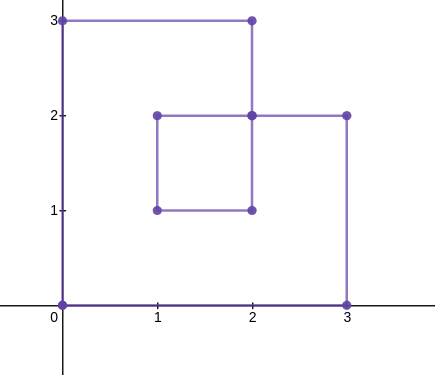#Boost C++ Libraries

...one of the most highly regarded and expertly designed C++ library projects in the world.

##### touches (one geometry)

Checks if a geometry has at least one touching point (self-tangency)

###### Synopsis

```template<typename Geometry>
bool touches(Geometry const & geometry)```

###### Parameters

Type

Concept

Name

Description

Geometry const &

Any type fulfilling a Geometry Concept

geometry

A model of the specified concept

###### Returns

Returns true if the geometry is self-touching

Either

`#include <boost/geometry.hpp>`

Or

`#include <boost/geometry/algorithms/touches.hpp>`

###### Conformance

The function touches implements function Touches from the OGC Simple Feature Specification.

The version with one parameter is additional and not described in the OGC standard

NoteImplemented for Point/Linestring/MultiLinestring/Polygon/MultiPolygon.
###### Examples

Checks if a geometry has at least one touching point (self-tangency)

```#include <iostream>

#include <boost/geometry.hpp>
#include <boost/geometry/geometries/point_xy.hpp>
#include <boost/geometry/geometries/polygon.hpp>

namespace bg = boost::geometry;int main()
{
// Checks if the geometry has self-tangency.
bg::model::polygon<bg::model::d2::point_xy<double> > poly1;
bg::read_wkt("POLYGON((0 0,0 3,2 3,2 2,1 2,1 1,2 1,2 2,3 2,3 0,0 0))", poly1);
bool check_touches = bg::touches(poly1);
if (check_touches) {
std::cout << "Touches: Yes" << std::endl;
} else {
std::cout << "Touches: No" << std::endl;
}

bg::model::polygon<bg::model::d2::point_xy<double> > poly2;
bg::read_wkt("POLYGON((0 0,0 4,4 4,4 0,2 3,0 0))", poly2);
check_touches = bg::touches(poly2);
if (check_touches) {
std::cout << "Touches: Yes" << std::endl;
} else {
std::cout << "Touches: No" << std::endl;
}

return 0;
}
```

 Convenient namespace alias

Output:

```Touches: YesTouches: No
```